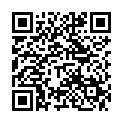## Y5 Number - Fractions (including Decimals and Percentages) - Interactive Quiz

### Related Worksheets

An interactive quiz which test your understanding of all the Fractions (including Decimals and Percentages) objectives in the Year 5 curriculum. Choose one objective or multiple objectives. You can save, or print, your test results as a pdf at the end of the quiz. Ideal for formative or summative assessment.

Alternatively, you can use the Interactive Maths Quiz which includes objectives from all strands of the Year 5 curriculum.

This quiz tests the following objectives:

• compare and order fractions whose denominators are all multiples of the same number
• identify, name and write equivalent fractions of a given fraction, represented visually, including tenths and hundredths
• recognise mixed numbers and improper fractions and convert from one form to the other and write mathematical statements > 1 as a mixed number [for example,+= 1]
• add and subtract fractions with the same denominator, and denominators that are multiples of the same number
• multiply proper fractions and mixed numbers by whole numbers, supported by materials and diagrams
• read and write decimal numbers as fractions [for example, 0.71 =]
• recognise and use thousandths and relate them to tenths, hundredths and decimal equivalents
• round decimals with 2 decimal places to the nearest whole number and to 1 decimal place
• read, write, order and compare numbers with up to 3 decimal places
• solve problems involving number up to 3 decimal places
• recognise the per cent symbol (%) and understand that per cent relates to ‘number of parts per 100’, and write percentages as a fraction with denominator 100, and as a decimal fraction
• solve problems which require knowing percentage and decimal equivalents ofand those fractions with a denominator of a multiple of 10 or 25Scan to open this game on a mobile device. Right-click to copy and paste it onto a homework sheet.